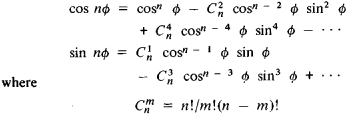# De Moivre's Theorem

Also found in: Wikipedia.

## De Moivre's theorem

[də′mwäv·rəz ‚thir·əm]
(mathematics)
The n th power of the quantity cos θ + i sin θ is cos n θ + i sin n θ for any integer n.
McGraw-Hill Dictionary of Scientific & Technical Terms, 6E, Copyright © 2003 by The McGraw-Hill Companies, Inc.
The following article is from The Great Soviet Encyclopedia (1979). It might be outdated or ideologically biased.

## De Moivre’s Theorem

a rule for raising a complex number to the nth power when the number is expressed in polar form as

z; = p(cos φ + i sin φ)

According to the rule, the modulus ρ of the complex number is raised to the given power and the amplitude φ is multiplied by the exponent:

zn = [p(cos φ + i sin φ)]n

= pn(cos nφ + i sin nφ) The theorem was devised by A. De Moivre in 1707, and its modern form was proposed by L. Euler in 1748.

De Moivre’s theorem can easily be used to express cos nϕ and sin nϕ in terms of powers of cos φ and sin φ. If we set ρ = 1 in De Moivre’s theorem and separately equate the real and imaginary parts, then we getare binomial coefficients. Inversion of De Moivre’s theorem leads to a formula for extracting the root of a complex number.

References in periodicals archive ?
Traditionally, z is assumed to be a complex number and the roots are usually determined by using de Moivre's theorem adapted for fractional indices.
Students studying a mathematics specialism as a part of the Victorian VCE (Specialist Mathematics, 2010), HSC in NSW (Mathematics Extension in NSW, 1997) or Queensland QCE (Mathematics C, 2009) will be familiar with de Moivre's theorem and its applications to complex numbers (see the short discourse by Bardell (2014) for further details).
Now apply de Moivre's theorem using the fractional index 1/n to find the n-th roots of z.
A true plan view of the surface intersections A = B = 0 is shown in Figure 9, with the unit circle superimposed to indicate the solution found from using de Moivre's theorem. Finally, a section taken at H = 0 through surface A will reveal the original quintic equation as it would appear in the Cartesian x - y plane.
This paper describes how a simple application of de Moivre's theorem may be used to not only find the roots of a quadratic equation with real or generally complex coefficients but also to pinpoint their location in the Argand plane.
Now apply de Moivre's theorem, as expressed in Equation (3) with fractional indices.
These then are the two roots of the complex number 4-7.57 It is noted that de Moivre's theorem naturally anticipates results in the complex (Argand) plane.
Equation (5b) has been rendered in a standard form amenable to solution using de Moivre's theorem as expressed by Equation (3).
By applying de Moivre's theorem to each term on the right hand side of equation (2), I obtain three different branches of y and use them to show that the domain of the curve is the set of all real numbers.
Applying de Moivre's theorem then gives the following three distinct values of u and v respectively:
Applying de Moivre's theorem to equations (14a) and (14b) gives the following three distinct expressions (branches) for u and v respectively:
Applying de Moivre's theorem gives the following three distinct values of u and v respectively:
Site: Follow: Share:
Open / Close